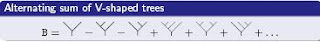## M Theory Lesson 97

F. Chapoton has a wonderful set of slides on operads and combinatorics. On slide 42 he explains how a symmetric operad $P$ (ones with a permutation action) in the category Set may be thought of as a Joyal species functor into Set (recall that species came up in Abel sum theory) along with an arrow $P.P \rightarrow P$ giving composition. Note that this is just how multiplication looks like for a monad.

On slide 62 we see a novel way of looking at the expression $J = 1 + 2J + J^2$, which arose in the blogrolling discussion. Chapoton’s solution for $J$ is a series of binary treeswhich has a kind of inverse with respect to an interesting tree operad, called OverUnder, due to A. Frabetti. This inverse is the alternating seriesNotice the analogy with Rota’s Mobius inversion, where alternating series naturally arise. It would be nice to understand alternation better, as Euler characteristics are defined using such series. In fact, there is a map from the OverUnder operad to the Associative operad which takes a sum of trees to the Abel series that collects sets of trees together. In this paper Frabetti looks at trees for QED renormalisation.